# Complete the following chemical reactions.

Question:

Complete the following chemical reactions.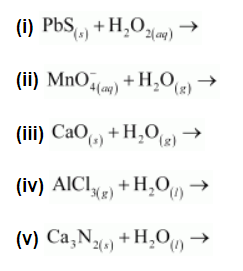Classify the above into (a) hydrolysis, (b) redox and (c) hydration reactions.

Solution: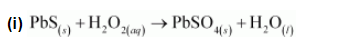H2O2 is acting as an oxidizing agent in the reaction. Hence, it is a redox reaction.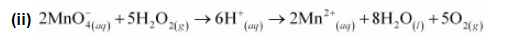$\mathrm{H}_{2} \mathrm{O}_{2(a q)}$ is acting as a reducing agent in the acidic medium, thereby oxidizing $\mathrm{MnO}_{4(a q)}^{-} .$Hence, the given reaction is a redox reaction.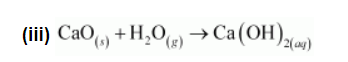The reactions in which a compound reacts with water to produce other compounds are called hydrolysis reactions. The given reaction is hydrolysis.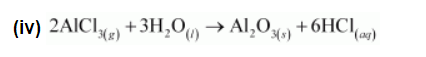The reactions in which a compound reacts with water to produce other compounds are called hydrolysis reactions. The given reaction represents hydrolysis of AlCl3.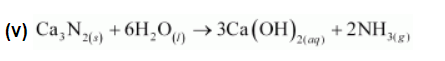The reactions in which a compound reacts with water to produce other compounds are called hydrolysis reactions. The given reaction represents hydrolysis of Ca3N2.